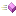The methods of the EllipticCurveCalculator class are listed here.

 Name DescriptionThis is Add, a member of class EllipticCurveCalculator.This is InverseMod, a member of class EllipticCurveCalculator.This is Modular, a member of class EllipticCurveCalculator.This is PointNeg, a member of class EllipticCurveCalculator.This is ScalarMult, a member of class EllipticCurveCalculator.